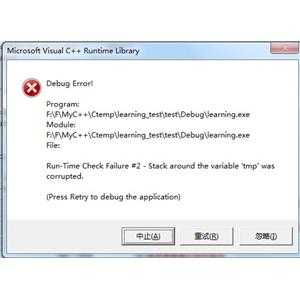﻿ Run-Time Check Failure #2 – Stack around the variable was corrupted | Code Bye

# Run-Time Check Failure #2 – Stack around the variable was corrupted

8年前 (2015-10-20) 908次浏览
```#define _CRT_SECURE_NO_DEPRECATE
#include<stdio.h>
#define MAX_SIZE 10
typedef struct _Que{
char value[MAX_SIZE];
} Que;
void initQ(Que * q)
{
char i;
for (i = 0; i < MAX_SIZE; i++)
{
q->value[i] = 0;
}
q->head = q->tail = q->count = 0;
}
char isEmpty(Que * q)
{
else	return 0;
}
char isFull(Que * q)
{
else	return 0;
}
char enQ(Que * q, char value)
{
if(isFull(q))	return 0;
q->count++;
return 1;
}
char deQ(Que * q, char * value)
{
if(isEmpty(q))	return 0;
*value = q->value[q->tail];
q->tail++;
if(q->tail==MAX_SIZE)	q->tail = 0;
q->count--;
return 1;
}
int main()
{
Que q;
char tmp,i;
initQ(&q);
for(i=0;i<4;i++)
{
scanf("%d", &tmp);
enQ(&q, tmp);
}

while(!isEmpty(&q))
{
deQ(&q, &tmp);
printf("%d\n", tmp);
}

return 0;
}```scanf(“%d”, &tmp); tmp明明是char类型却要充当int, 那句错误信息说得很清楚吧

tmp 是一个 char， 只有 1 个字节大小。 scanf(“%d”  中 %d 对应的是 int，它会访问传入的地址开始的 4 个字节的内容，你只有 1 个字节，访问就越界了。

CodeBye 版权所有丨如未注明 , 均为原创丨本网站采用BY-NC-SA协议进行授权 , 转载请注明Run-Time Check Failure #2 – Stack around the variable was corrupted

[1034331897@qq.com]